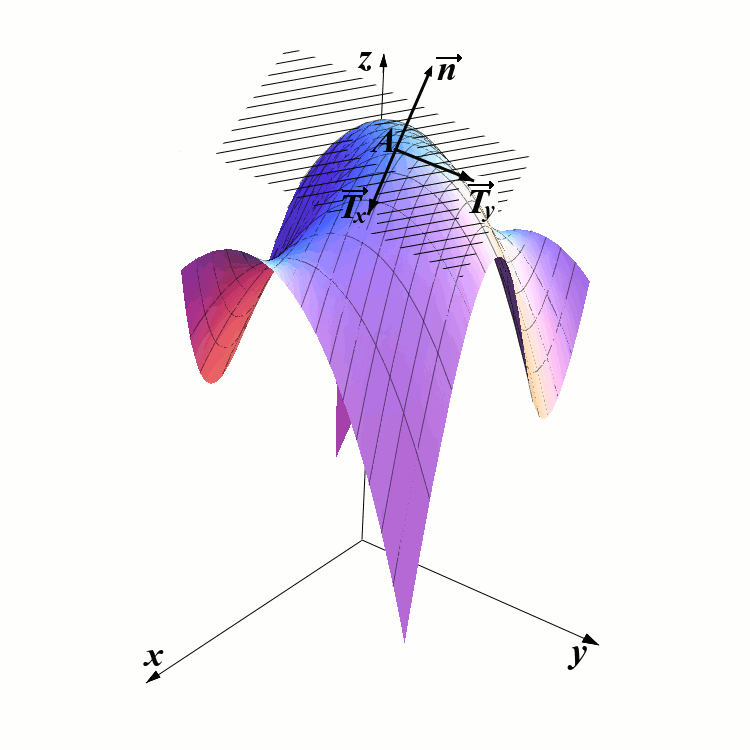# Tangent Planes

## Tangent planes to graphs of functions

Assume that $$f(x,y)$$ is a function of two variables. Its graph is a surface $$z=f(x,y)$$. Our goal is to find an equation of the tangent plane passing trough a point $$A(x_0,y_0,z_0)$$ on the surface.In the picture above, you can see a graph of a surface $$z=f(x,y)$$ and a point $$A$$ on that graph. Our goal is to find the equation of the shaded plane. In order to do this, we just need to find the coordinates of the normal vector $$\overrightarrow{n}$$.

Recall that in the single variable calculus, the quantity $$f^{\prime}(a)$$ represented the slope of the tangent line to the graph of $$f$$ at the point $$a$$. The tangent vector is then equal to $$\overrightarrow T=\langle 1, f^{\prime}(a)\rangle$$.

In multivariable calculus, the tangent to the surface is a plane, rather than a vector. The quantity $$f_x(x_0,y_0,z_0)$$ also represents a slope of a certain vector. This vector belongs to the tangent plane, and is parallel to the $$xz$$ plane. Let us call this vector $$\overrightarrow T_x$$. Let us find its coordinates, i.e. let us find real numbers $$p$$, $$q$$, and $$r$$ such that $\overrightarrow T_x=\langle p,q,r\rangle.$ First of all $$q$$ is easy to find. It is equal to $$0$$. The coordinates $$p$$ and $$r$$ are obtained in the same way as in single-variable calculus. We may take $$p=1$$ and $$r=f_x(x_0,y_0)$$. Therefore we have $\overrightarrow T_x(x_0,y_0)=\langle 1,0,f_x(x_0,y_0)\rangle.$ Similarly, we obtain another vector that belongs to the tangent plane: $\overrightarrow T_y(x_0,y_0)=\langle 0,1,f_y(x_0,y_0)\rangle.$ And now we use a magnificent trick: Normal vector can be obtained as a cross product of $$\overrightarrow T_x$$ and $$\overrightarrow T_y$$. Thus:

$\overrightarrow n(x_0,y_0)= \overrightarrow T_x\times\overrightarrow T_y=\left|\begin{array}{ccc} \overrightarrow i& \overrightarrow j & \overrightarrow k \newline 1 & 0 & f_x \newline 0 & 1 & f_y \end{array}\right|=\langle -f_x,-f_y,1\rangle.$ We have obtained the following theorem:

The Equation of the Tangent Plane If a surface is defined as a graph of $$z=f(x,y)$$, and if $$A(x_0,y_0,z_0)$$ is a point on the surface, then a normal vector to the tangent plane at the point $$A$$ is given by $\overrightarrow n(x_0,y_0)=\langle -f_x(x_0,y_0), -f_y(x_0,y_0),1\rangle.$ The equation of the plane tangent to the surface at the point $$A$$ is given by: $-f_x(x_0,y_0)(x-x_0)-f_y(x_0,y_0)(y-y_0)+(z-z_0)=0.$

Example 1 Consider the function: $f(x,y)=3-x^2-y^2-3x^3y+2xy.$ Find the equation of the tangent plane to the surface at the point $$M(0,1,2)$$.

Example 2 Find the equation of the tangent plane to the ellipsoid $$x^2+4y^2+9z^2=1$$ at the point $$\left(\frac12,\frac14,\frac{\sqrt 2}3\right)$$.

## Tangent planes to surfaces given by parametric equations

Assume that the surface $$S$$ is given by its parametric equations: \begin{eqnarray*} x&=& X(s,t)\\ y&=& Y(s,t)\\ z&=&Z(s,t), \end{eqnarray*} where $$X$$, $$Y$$, and $$Z$$ are differentiable functions of two variables. Assume that we want to find the tangent plane to the surface $$S$$ at the point $$(x_0,y_0,z_0)$$. Since each point of $$S$$ is obtained when a particular pair $$(s,t)$$ is plugged into the equations above, we may assume that there exists a pair $$(x_0,y_0)$$ such that $$(x_0,y_0,z_0)=(X(s_0,t_0),Y(s_0,t_0),Z(s_0,t_0))$$. Consider the function $$\overrightarrow R(s,t)=\langle X(s,t), Y(s,t), Z(s,t)\rangle$$.

Theorem The normal vector to the tangent plane of $$S$$ at the point $$(x_0,y_0,z_0)$$ is parallel to the vector $\overrightarrow N_0= \frac{\partial }{\partial s}\overrightarrow R(s_0,t_0)\times \frac{\partial }{\partial t}\overrightarrow R(s_0,t_0).$

We will now solve the same problem as in Example 2 by parametrizing the ellipsoid.

Example 3 Find the equation of the plane tangent to the ellipsoid $$x^2+4y^2+9z^2=1$$ at the point $$\left(\frac12,\frac14,\frac{\sqrt 2}3\right)$$.

## Practice problems

Problem 1. Find the tangent plane at $$(3,4,7)$$ to the surface given by its parametric equations: \begin{eqnarray*} x&=&5\cos s\\ y&=&5\sin s\\ z&=& t\\ 0\leq&s&\leq 2\pi\\ -\infty < &t& < +\infty. \end{eqnarray*}
• (A) $$x+y+z=5\cos s+5\sin s+t$$
• (B) $$3(x-5\cos s)+4(y-5\sin s)+(z-t)=0$$
• (C) $$z=5x+5y$$
• (D) $$4(x-3)+3(y-4)=0$$
• (E) $$3x+4y=25$$

Problem 2. Consider the surface given by the equation $$z=7-x-y$$. Find the tangent plane of this surface at the point $$(x_0,y_0)=(1,-3)$$.
• (A) $$z=7-x-y$$
• (B) $$3(x+1)+(y+3)+z=0$$
• (C) $$3(x-1)+(y-3)+z-2=0$$
• (D) $$z=x+2y+3$$
• (E) $$x+y+z=1$$

Problem 3. Find the tangent plane to the sphere $$x^2+y^2+z^2=9$$ at the point $$\left(1,2,-2\right)$$.

Problem 4. Find the tangent plane to the ellipsoid $$\frac{x^2}{196}+y^2+z^2=1$$ at the point $$\left(4,\frac37,\frac67\right)$$.

Problem 5. Assume that $$S$$ is a surface and $$M$$ a point on $$S$$. Assume that $$\alpha$$ is a plane tangent to $$S$$ at the point $$M$$ and assume that $$\overrightarrow a$$ and $$\overrightarrow b$$ are two vectors in the plane $$\alpha$$. Consider the following mathematical propositions:
• (I) The vector $$\overrightarrow a\times \overrightarrow b$$ is also a vector in the tangent plane $$\alpha$$.
• (II) The vector $$\overrightarrow a+\overrightarrow b$$ is also a vector in the tangent plane $$\alpha$$.
• (III) The vector $$\overrightarrow a\times \overrightarrow b$$ is a vector normal to the tangent plane $$\alpha$$.
Which of the following is true?
• (A) The propositions (I) and (II) are true for every surface $$S$$.
• (B) The propositions (I) and (III) are true for every surface $$S$$.
• (C) The propositions (II) and (III) are true for every surface $$S$$.
• (D) All three propositions (I), (II), and (III) are true for all surfaces $$S$$.
• (E) There is a surface $$S$$ for which none of the above propositions is true.